# Introduction

## Distance Formula

The distance between two points P(x1, y1) and Q(x2, y2), is given

by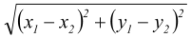.

### Section Formula

If R divides the join of P(x1, y1) and Q(x2, y2) in the ratio m : n (m, n > 0), then

x =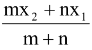y =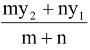(Internal Division)

and x =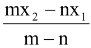y =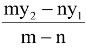(External Division)

## Area of a Triangle

The area of a triangle ABC with vertices A(x1, y1), B(x2, y2) and C(x3, y3) denoted conventionally by the symbol Δ is given by

Δ =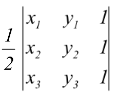=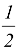{x1(y2 − y3) + x2(y3 − y1) + x3(y1 − y2)}

I. If one vertex say C(x3, y3) is the origin (0, 0) then Δ =|x1y2 − x2y1|.

II. If a1x + b1y + c1 = 0, a2x + b2y + c2 = 0 and a3x + b3y + c3 = 0 are the sides of a triangle then the area of a triangle is given by

Δ =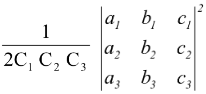where C1 C2 C3 are the cofactors of c1 c2 c3 in the determinant.